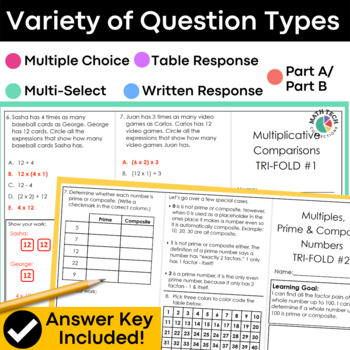# 4th Grade Measurement, Area & Perimeter, Measure Angles - 4.MD.1 - 4.MD.7Subject
Resource Type
Format
Zip (23 MB|42 pages)
Standards
\$10.50
\$10.50

#### Also included in

1. These math tri-folds are perfect for you to use during your guided math groups, math assessments, or homework. Three tri-folds per standard give you plenty material to introduce, practice, and assess all 4th grade math standards. This resource has been updated to include a Google Slides version with
\$25.00
\$40.00
Save \$15.00
2. This fourth grade math bundle includes 3 AMAZING MATH RESOURCES! Included: Math Tri-Folds, Math Sorts, and Math Morning Work!This is a MUST have resources for your 4th grade classroom. The resources included are flexible and may be used for morning work, whole group practice, guided math practice, i
\$67.50
\$118.50
Save \$51.00
3. This 4th grade math bundle includes math tri-folds that are intended for use during small group instruction and Google Forms quizzes that can be used as pre and post math assessments. This 4th grade math practice bundle includes both PRINT and DIGITAL format. - The printable format includes 84 bookl
\$40.00
\$80.00
Save \$40.00

### Description

These 4th grade math tri-folds are perfect for you to use during your guided math groups, math assessments, or homework. Three tri-folds per standard give you plenty material to introduce, practice, and assess!

This resource has been updated to include a Google Slides version with simple text boxes.

These math booklets are organized by standard, so you will save time planning your small group lessons.

STANDARDS COVERED: Measurement & Data

21 tri-folds (3 per standard)

4.MD.1: I can show that I know the relative sizes of measurement units within one system of units. I can show the measurements of a larger unit in terms of a smaller unit and record these in a table.

4.MD.2: I can use the four operations to solve word problems involving measurement, including problems involving simple fractions or decimals. I can represent the measurements on a number line.

4.MD.3: I can use what I know about area and perimeter to solve real world problems involving rectangles.

4.MD.4: I can make a line plot to display a data set of measurements in fractions of a unit. I can solve problems involving addition and subtraction of fractions by using the information presented in line plots.

4.MD.5: I can recognize angles as geometric shapes that are formed when two rays share a common endpoint.

4.MD.6: I can measure angles in whole-number degrees using a protractor. I can sketch angles of specified measures.

4.MD.7: I can solve addition and subtraction problems involving angles.

* Editable small group lesson plan page

* Editable level of mastery pages

* EDITABLE WORD format of each tri-fold

Ways to use in your classroom: whole group, small group, math centers, homework, math quiz, math test, math review, math study guide

MORE MATH TRI-FOLDS

3rd Grade - Written Response Tri-Folds

4th Grade - Written Response Tri-Folds

5th Grade - Written Response Tri-Folds

Total Pages
42 pages
Included
Teaching Duration
Lifelong tool
Report this Resource to TpT
Reported resources will be reviewed by our team. Report this resource to let us know if this resource violates TpT’s content guidelines.

### Standards

to see state-specific standards (only available in the US).
Recognize angle measure as additive. When an angle is decomposed into non-overlapping parts, the angle measure of the whole is the sum of the angle measures of the parts. Solve addition and subtraction problems to find unknown angles on a diagram in real world and mathematical problems, e.g., by using an equation with a symbol for the unknown angle measure.
Measure angles in whole-number degrees using a protractor. Sketch angles of specified measure.
An angle that turns through 𝘯 one-degree angles is said to have an angle measure of 𝘯 degrees.
An angle is measured with reference to a circle with its center at the common endpoint of the rays, by considering the fraction of the circular arc between the points where the two rays intersect the circle. An angle that turns through 1/360 of a circle is called a “one-degree angle,” and can be used to measure angles.
Recognize angles as geometric shapes that are formed wherever two rays share a common endpoint, and understand concepts of angle measurement: